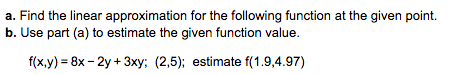# a. Find the linear approximation for the following function at the given pointb. Use part (a) to estimate the given function value.f(x,y)-8x-2y + 3xy; (2,5);estimate f(1.9.4.97)

Questionhelp_outlineImage Transcriptionclosea. Find the linear approximation for the following function at the given point b. Use part (a) to estimate the given function value. f(x,y)-8x-2y + 3xy; (2,5); estimate f(1.9.4.97) fullscreen
check_circleExpert Solution
Step 1
• We have how to find linear approximation of f(x) at (2 ,5) and Then approximate the value Of function f(x) at x=1.9 and y=4.97.

• Information is given below
Step 2
• We will use following rules to find derivative and linear approximation of f(x,y).

• Rules are given below:
Step 3
• Now with the help of about derivative rules and linear approximation formula we will find Linear approximation of f(x, y) at (2, 5).

• Af...

### Want to see the full answer?

See Solution

#### Want to see this answer and more?

Solutions are written by subject experts who are available 24/7. Questions are typically answered within 1 hour*

See Solution
*Response times may vary by subject and question
Tagged in

### Calculus